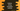# Python string isnumeric method## Python string isnumeric method:

The isnumeric method returns a boolean value and this method is used to check if all characters of a string are numeric characters or not in Python. Let me show you how this method works with examples.

### Python string isnumeric method definition:

The isnumeric method is defined as like below:

``str.isnumeric()``

This method returns True if all characters in the string are numeric characters. It returns False otherwise. It also returns False if the string doesn’t contain at least one character.

A character is called a numeric character if the Numeric_Type is Digit, Decimal or Numeric for that character.

If all characters have unicode numeric value property, or digit characters, these are considered as numeric.

### Example of isnumeric:

Let’s learn how isnumeric works with an example:

``````given_str = ['1', '2', '10', '11.23', '-123', '+123', '', '0', '2/3', '2²']

for item in given_str:
print(f'{item} => {item.isnumeric()}')``````

Here, given_str is an array of different types of strings. The for loop iterates through the elements of this array one by one and prints the result of isnumeric() for each.

If you run this program, it will print:

``````1 => True
2 => True
10 => True
11.23 => False
-123 => False
+123 => False
=> False
0 => True
2/3 => False
2² => True``````

It returns False if it holds +, -, ., / etc. symbols and even for an empty string.

Also, for characters like ½, ², it returns True.

``````given_str = ['²', '½']

for item in given_str:
print(f'{item} => {item.isnumeric()}')``````

It will print True for both.

### Example of isnumeric with unicode values:

We can also use unicode values with isnumeric. If it represents any numeric value, it will return true.

For example, \u00B2 is the unicode for ². If we use it with isnumeric, it will return True:

``print('\u00B2'.isnumeric())``

### Check if an user input value is numeric or not using isnumeric:

Let’s check if an user-input value is numeric or not by using isnumeric method:

``````s = input('Enter a value: ')

if s.isnumeric() == True:
print('It is a numeric value')
else:
print('It is not a numeric value')``````

The user input value is stored in s and we are using isnumeric() method on it to check if it is numeric or not. It will print output as like below:

``````Enter a value: 123
It is a numeric value

Enter a value: +123
It is not a numeric value

Enter a value: -123
It is not a numeric value

Enter a value: 1.23
It is not a numeric value

Enter a value: hello
It is not a numeric value

Enter a value: 123456778901
It is a numeric value

Enter a value: 000000
It is a numeric value

Enter a value:
It is not a numeric value``````